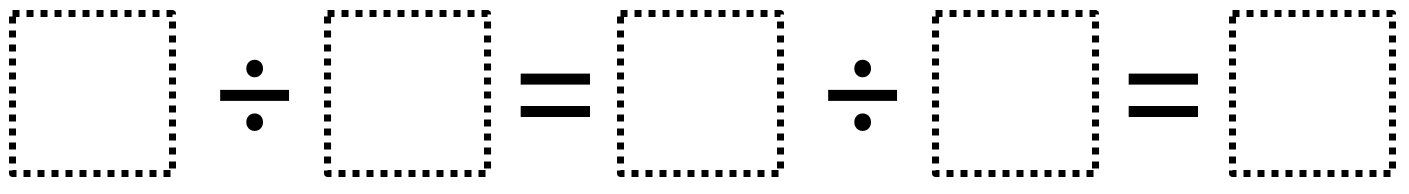Home > Grade 4 > Whole Number Division

# Whole Number Division

Directions: Using the digits 1 to 9 at most one time each, fill in the boxes to make a true statement.### Hint

What possible values can the single digit have?

The only possible answer is 8 ÷ 4 = 6 ÷ 3 = 2

Source: Owen Kaplinsky

## Create A Pattern

Directions: Use the digits 0 to 9, at the most one time each, place a …

1.This really puzzled my students. When we looked at the hint and then the answer, they realized there was a mistake. 8 divided by 4 is 2, not 6.

2.Thanks! What I meant to say was 8/4=2 and 6/3=2.

•That still doesn’t make a true statement, though. Is there something I’m not understanding?

•I think so. Instead of 8/4=2 and 6/3=2, you could say 8/4=6/3=2. Also, you can think of it as three parts. Each one equals the same thing. Part one is 8/4 or 2, part 2 is 6/3, or 2, and part 3 is 2.

3.Some parentheses might help

4.i think it’s 8 4 6 3 2

5.i think it’s 8 4 6 3 2

6.8 divided by 2=4 divided by 2=2

7.i think it might be 8 4 6 3 2

8.i did it wrong its 8 divided by 4 = 2 so the answer is 2

9.Person aka kaleya

8 ÷ 4 = 6 ÷ 3 = 2

10.8 ÷ 4 = 6 ÷ 3 = 2

11.JaliyahSingleton

8 ÷ 4 = 6 ÷ 3 = 2

12.it should be 8/4=2/1=1 because 8/4=6/3=2 and 8/4=2/3=2 cant be true

13.8 ÷ 4 = 6 ÷ 3 = 2 is the answer

14.8 divide 4=6 divide 3=2

15.8/4=6/3=2

16.Perla Martinez

8/4=6/3=6

17.8/4=6/3=2

I made an error the first time with 8/4 = 2/1 = 2 but realized I used 2 twice.

18.lavellas peterson

8/4=6/3=2

19.I am wondering if posting it like this would provide some clarification:
_ / _= _ / _

Then, the answer would be 8/4=6/2.

Couldn’t 8/4=2/1 also be an answer?

•Yeah, that could be another answer.

20.I allowed them to put 2 digits in a line if they wanted to. This helped.

54/9 = 6/3 = 2